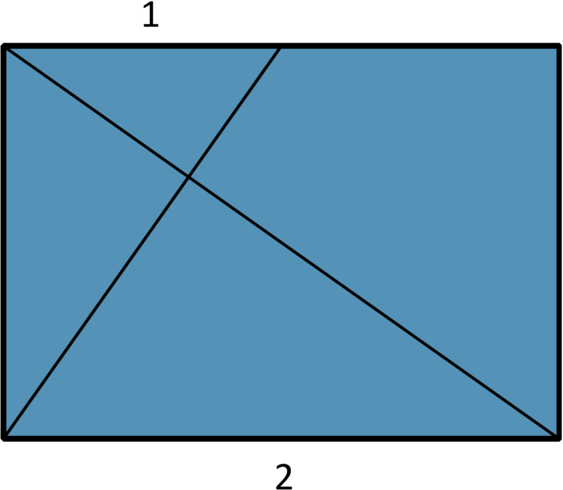# 4. Dividing lengths into equal parts

What’s half of three quarters? With a piece of paper in your hands, it’s easy to show that half of three quarters is three eighths. As folding in half is easy, you should be able to divide the edge of a rectangle into quarter, eighths, sixteenths, etc.

However, dividing into thirds, fifths and other odd fractions is less easy. With fractions like fifteenths, it’s probably easier to divide into sixteenths and remove one sixteenth. The most practical solution is to measure and calculate. However, dividing lengths into thirds can be quicker without any tools, except your brain and hands. One method is to curl the paper into an S shape and adjust until each part is the same length.

There are many exact ways of dividing into thirds. In the previous part, I asked how you could use the 60 degree fold to divide an edge into thirds. This is one way:Figure 4-1 The intersection of the two lines is one third along and one third down from the top left. The proof is by looking and focusing on the similar triangles.

Here is one of the simplest ways of trisecting a length. This is a proof by looking that requires you to understand “similarity”. Two triangles are similar if you can scale, rotate and translate one into the other. Stretching, skewing and other distortions are not allowed. Two triangles that have the same lengths and angles are “congruent”: identical, in everyday language.Figure 4-2 (I) Guess one third, x. (ii) Fold the right part in half. (iii) Then Fold the new left part in half. (iv) Repeat and the folds will converge (Fujimoto)

Other proofs have been written e.g.

These give some other interesting methods, some of which are listed in Dividing a square into thirds. Most methods are surprisingly simple to fold but it can be challenging to find the simplest and most convincing proof.Dividing a square into thirds: Here are eight methods for dividing a square into thirds. How can you prove each method works? What are the advantages and disadvantages of each method? Which methods can be generalised to oblongs and other divisions e.g. fifths and sevenths?

Fujimoto’s iterative method is unusual.

You can see that x quickly converges to 0.333. The better the first guess, the quicker the convergence. For an edge of 20cm, the error for a very bad first fold 10 cm is less than 1mm after six iterations.

 1 0.5 0.4 2 0.25 0.3 3 0.375 0.35 4 0.313 0.325 5 0.344 0.338 6 0.328 0.331 7 0.336 0.334 8 0.332 0.333 9 0.334 0.334 10 0.333 0.333

With a little variation, this method can produce fifths and other divisions. Can you see how?